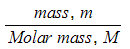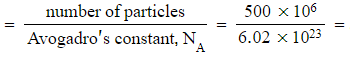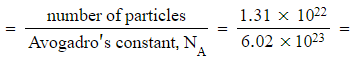# Edexcel A Level Chemistry:复习笔记1.6.4 The Mole & the Avogadro Constant

### The Mole & Avogadro

• The Avogadro constant (NA or L) is the number of particles equivalent to the relative atomic mass or molecular mass of a substance
• The Avogadro constant applies to atoms, molecules, ions and electrons

• The value of NA is 6.02 x 1023 g mol-1
• The mass of a substance with this number of particles is called a mole (mol)
• This can be called the molar mass
• This is the mass of substance that contains the same number of fundamental units as exactly 12.00g of carbon-12
• The amount / number of moles of a substance, n, the mass of the substance, m, and the molar mass, M, are linked by the equation:

n =• One mole of any element is equal to the relative atomic mass of that element in grams
• If you had one mole of carbon in your hand, that is 6.02 x 1023 atoms of carbon, you would have a mass of 12.00 g
• One mole of water would have a mass of (2 x 1 + 16) = 18 g

#### Worked Example

1. What is the molar mass of water?
2. How many moles are there in 100 g of water?
3. How many water molecules are there in 100 g of water?

1. Molar mass of water, H2O = (2 x 1.0) + 16.0 = 18.0 g mol-1
2. Molesmoles (to 3 s.f.)
3. Number of molecules = number of moles x Avogadro's constant = 5.56 x (6.02 x 1023) = 3.35 x 1024 molecules

#### Worked Example

What is the mass of the following:

1. Five hundred million atoms of platinum
2. (1.31 x 1022) molecules of ethanol

Number of moles8.31 x10-16 moles

Mass = moles x molar mass = (8.31 x10-16) x 195.1 = 1.62 x 10-13 g (lots of atoms, a tiny mass)

Molar mass of ethanol, C2H5OH = (2 x 12.0) + (5 x 1.0) + 16.0 + 1.0 = 46.0 g mol-1

Number of moles0.0218 moles

Mass = moles x molar mass = 0.0218 x 46.0 = 1.00 g (an exceptionally large number of molecules in just 1 g)

#### Exam Tip

• When you are completing calculations using Avogadro's constant, you may end up with answers that seem very large or very small - don't automatically assume that they must be wrong
• Remember, Avogadro's constant is a VERY large number:
• 6.02 x 1023 or 602 000 000 000 000 000 000 000
• So when you multiply or divide by Avogadro's constant, your answers will, naturally, become very large or very small